Courses

# Test: Chemistry- 4

## 45 Questions MCQ Test NEET Mock Test Series | Test: Chemistry- 4

Description
This mock test of Test: Chemistry- 4 for NEET helps you for every NEET entrance exam. This contains 45 Multiple Choice Questions for NEET Test: Chemistry- 4 (mcq) to study with solutions a complete question bank. The solved questions answers in this Test: Chemistry- 4 quiz give you a good mix of easy questions and tough questions. NEET students definitely take this Test: Chemistry- 4 exercise for a better result in the exam. You can find other Test: Chemistry- 4 extra questions, long questions & short questions for NEET on EduRev as well by searching above.
QUESTION: 1

Solution:
QUESTION: 2

Solution:
QUESTION: 3

### In the following compounds,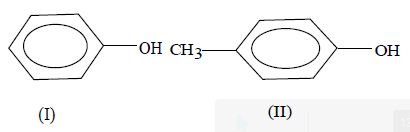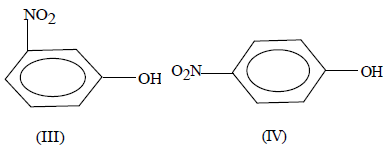The order of acidity is

Solution:
QUESTION: 4
Which of the following is a Riemer-Tiemann reaction
Solution:
QUESTION: 5
Victor Meyer’s test is not given by
Solution:
QUESTION: 6
When phenol is treated withe excess bromine water
Solution:
QUESTION: 7
n-Propyl alcohol and isopropyl alcohol can be chemically distinguished by which reagent
Solution:
QUESTION: 8
Glycerol is treated with excess of HI at room temperature and then with more HI at 426K. The
final product obtained is
Solution:
QUESTION: 9

Phenol reacts with PCl5 to give mainly

Solution:

Solution :

Phenyl reacts with PCl5 to give only a small amount of chlorobenzene, the main product is triphenyl phophate, (C6H5)3PO4, the ester of phosphoric acid. With PCl3, we get ester of phosphoric acid, (C6H5)3PO3.

QUESTION: 10
In the reaction of phenol with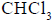and aqueous NaOH at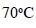(343 K), the electrophile attacking the ring is
Solution:
QUESTION: 11
Which of the following on oxidation gives a ketone ?
Solution:
QUESTION: 12
Dow’s reaction involves
Solution:
QUESTION: 13
Correct increasing order of acidity is as follows ?
Solution: D is correct because increasing order of replaceable hydrogen.
QUESTION: 14
Which of the following cannot be made by using Williamson’s synthesis ?
Solution:
QUESTION: 15
The compound obtained by the reaction of ethene with diborane followed by hydrolysis with alkaline
hydrogen peroxide is
Solution:
QUESTION: 16
When benzenediazonium chloride is treated with water, the compound formed is
Solution:
QUESTION: 17
Which one of the following compounds will be most readily attacked by an electrophile ?
Solution:
QUESTION: 18
The alcohol which does not give a stable compound on dehydration is
Solution:
QUESTION: 19
What amount of bromine will be required to convert 2g of phenol into 2,4, 6-tribromophenol ?
Solution:
QUESTION: 20
The reaction of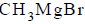with acetone and hydrolysis of the resulting product gives
Solution:
QUESTION: 21
Identify X in the following sequence of reaction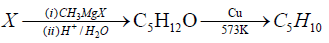Solution:
QUESTION: 22
Which of the following compounds will react with two moles of?
Solution:
QUESTION: 23
Ethyl ethanoate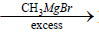P.The product P will be
Solution:
QUESTION: 24
Which of the following pairs of solutions is isotonic ?
Solution:
QUESTION: 25
At 298K, highest osmotic pressure is exhibited by a 0.1M solution of
Solution:
QUESTION: 26
Which of the following is an electrically prepared semipermeable membrane ?
Solution:
QUESTION: 27
What will happen if an egg whose white surface is removed by dilute HCl solution is placed in a saturated solution of NaCl ?
Solution:
QUESTION: 28
The van’t Hoff factor for a 0.1 M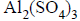solution is 4.20. The degree of dissociation is
Solution:
QUESTION: 29
The molar boiling-point constant for water is 0.513 Km–1. When 0.1 mole of sugar is dissolved in 200.0 g of water, the solution boils under a pressure of 1.0 atm at
Solution:
QUESTION: 30
When mercuric iodide is added to an aqueous solution of potassium iodide (in excess), a soluble complex,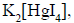is formed. Then,
Solution:
QUESTION: 31
The degree of association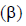is given by the expression
Solution:
QUESTION: 32
The average osmotic pressure of human blood is 7.8 bar at 37oC. What is the concentration of an aqueous NaCl solution that could be introduced in the bloodstream?
Solution:
QUESTION: 33
Which of the following ions has smallest radii ?
Solution:
QUESTION: 34
The yellow colour of chromates changes to orange on acidification due to formation of
Solution:
QUESTION: 35
Which of the following is an acidic oxide ?
Solution:
QUESTION: 36
Malachine is a mineral of
Solution:
QUESTION: 37
The flux used in smelting of copper ores is
Solution:
QUESTION: 38
In the reaction between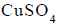and KI, a white precipitate is obtained. The precipitate has the
composition
Solution:
QUESTION: 39
An alloy which does not contain copper, is
Solution:
QUESTION: 40
Lucas reagent is
Solution:
QUESTION: 41
The carbon content in steel is
Solution:
QUESTION: 42
In the dichromate dianion
Solution:
QUESTION: 43
Arrange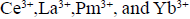in increasing order of their ionic radii
Solution:
QUESTION: 44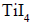on heating gives
Solution:
QUESTION: 45
Transuranic elements begin with
Solution: